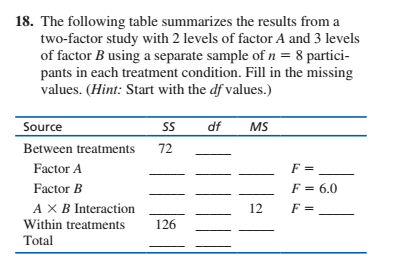# 18. The following table summarizes the results from atwo-factor study with 2 levels of factor A and 3 levelsof factor B using a separate sample of n = 8 partici-pants in each treatment condition. Fill in the missingvalues. (Hint: Start with the df values.)dfSourceSSMSBetween treatments72Factor AF = 6.0Factor BA X B InteractionWithin treatmentsTotal12F =126

Question
1 viewshelp_outlineImage Transcriptionclose18. The following table summarizes the results from a two-factor study with 2 levels of factor A and 3 levels of factor B using a separate sample of n = 8 partici- pants in each treatment condition. Fill in the missing values. (Hint: Start with the df values.) df Source SS MS Between treatments 72 Factor A F = 6.0 Factor B A X B Interaction Within treatments Total 12 F = 126 fullscreen
check_circle

Step 1

The degree of freedom for factor A = a – 1 = 2 – 1 = 1

The degree of freedom for factor B = b – 1 = 3 – 1 = 2

The degree of freedom for interaction A × B = (a - 1)× (b - 1) = 1 × 2 = 2

The degree of freedom between treatments = df A + df B + df (A × B) = 1+2+2 = 5

The degree of freedom within = ab(n - 1) = (2×3)(8 - 1) = 42

The total degree of freedom = abn – 1 = (2×3×8) – 1 = 47

Step 2

The values can be obtained as:

Step 3

Now,

...

### Want to see the full answer?

See Solution

#### Want to see this answer and more?

Solutions are written by subject experts who are available 24/7. Questions are typically answered within 1 hour.*

See Solution
*Response times may vary by subject and question.
Tagged in

### Statistics